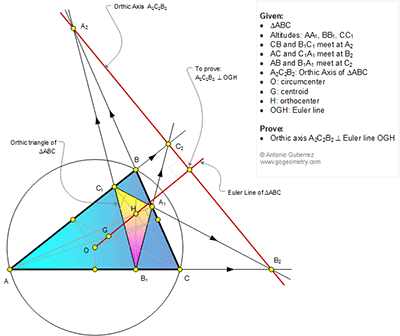## Friday, July 8, 2016

### Geometry Problem 1233: Triangle, Euler Line, Orthic Axis, Perpendicular, 90 Degrees, Orthic Triangle

Geometry Problem. Post your solution in the comment box below.
Level: Mathematics Education, High School, Honors Geometry, College.

Click the figure below to view more details of problem 1233.1.https://goo.gl/photos/hm7ruYYeKsGei8867

Draw 9 points circle of triangle ABC. The center N of this circle will be on Euler line NGH.
This circle will pass through A1 , B1, C1, and midpoints of sides of triangle ABC.
Draw new circle diameter ẠC
From B2 draw a tangent line to circle O1 at D.
Since( A,C, B1, B2)= -1 => DB1 ⊥ AC
Power of B2 to circle O= B2C . B2A=BD^2
But BD^2= B2B1. B2O1 (relationship in right triangle)= power of B2 to circle N
So B2 is on the radial axis of circles N and Ọ
Similarly C2 and A2 are on radial axis of circles N and O
Radial axis A2C2B2 will perpendicular to line connected centers of circles O and N

2.https://goo.gl/photos/AJ3pAb2CxNFo9j26A
New improved solution to problem 1233
Draw nine- points circle of triangle ABC. The center N of this circle will be on Euler line NGH.
This nine- points circle will pass through A1 , B1, C1, and midpoints of sides of triangle ABC.
Quadrilateral ACA1C1 is cyclic => B2C. B2A=B2A1. B2C1 (power of B2 to circle ACA1C1= power of B2 to circle O= power of B2 to circle N)
So B2 have the same power to both circles O or N => B2 will be on the radical axis of circles O and N.
Similarly ABA1B1 and BCB1C1 are cyclic and C2B.C2A=C2A1. C2B1 ,A2B.A2C= A2C1. A2B1 => C2 and A2 are on radical axis of circles N and O
Radical axis A2C2B2 will perpendicular to line connected centers of circles O and N